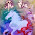## Monday, October 3, 2011

### Model Exploration using K-sample Plot in Big Data

Generically, error rate of predicting binary variable by a certain model becomes plateau increasing sample size. When the model fits training data , the error rate gains from 0 to true error. And when the model fits test data, the error rate decreases from 1 to true error. These phenomenons also occur predicting continuous variable, now error rate changes to proportion of explained variance. This figure clearly shows this concept (listed in textbook "Data Mining and Statistics for Decision Making").

When we have to deal with Big Data, most of all machine learning methods may not be able to calculate parameters because these calculation take to huge time. So I propose that checking performance of a certain model using
"K-sample Plot (K's plot)" explained below at times like dealing Big Data.

K's plot is simple algorithm drawing 1 - AUC (or proportion of explained variance) vs. sample size plot. The reason of using 1 - AUC instead of error rate, AUC can considerate sensitivity and specificity simultaneously.

Step 1. Sampling K observations from Big Data.
Step 2. Estimate 1 - AUC of training samples and test samples by Cross-Variation.
Step 3. Changing sampling number K from small to efficient size and calculate Step2.
Step 4. Plot K (x-axis) vs. 1 - AUC (y-axis).

I implement K's plot in R package named "KsPlot". Partial example code of KsPlot is this.

```library(KsPlot)
set.seed(1)
x1   <- rnorm(1000000)
set.seed(2)
x2   <- rnorm(1000000)
set.seed(3)
y    <- 2*x1 + x2**2 + rnorm(1000000)

X1      <- data.frame(x1 = x1, x2 = x2)
X2      <- data.frame(x1 = x1, x2 = x2, x3 = x2**2)
y       <- y

set.seed(1)
KsResult1 <- KsamplePlot(X1, y)
set.seed(1)
KsResult2 <- KsamplePlot(X1, y, Method="svm")
```

This example data have continuous outcome y and two continuous explanatory variables x1 and x2. Observation number of this data is 1 million!! Relationships of y and x1 is linear and y and x2 is quadratic. And If someone try to fitting linear model and SVM (support vector machine), calculation time of linear model is a few second but time of SVM is over 1 day!! So it's better that we can check performances of linear and SVM using K's plot.

Result of Linear Model.

Result of SVM.

Compare two results, we understand SVM is better than linear model in this case. At the present I implement these models (specified "Method=" option): linear model (lm), Support Vector Machine (svm), Newral Network (nn), is Random Forest (rf), Multipe Adaptive Regression Splines (mars), Classification and Regression Tree (cart) and LASSO (lasso) for continuous target variable. And implement lm and svm for binary target.

This method is some part of my doctor paper in Japanese. Now we are translating doctor paper in English and will try to publish to statistical journal.

1.Hi! Thanks for good article.
I think, Step2 can be executed as parallel on multicore or multi nodes, it can reduce running time.

2.It's true that calculate time become shorten by parallel or distributing process.
When maximum K is 1,000 the total calculate time is about a few (lm) or 10 second (svm). So if we use parallel process, it's time may become less than one second.

3.Very nice article this would definitely help the beginners, coding made easy with the help of example you shared. Big Data Training in Chennai
Big Data Training Institute in Chennai

4.Thanks for such a great article here. I was searching for something like this for quite a long time and at last I’ve found it on your blog. It was definitely interesting for me to read about their market situation nowadays.Well written article Thank You for Sharing with Us pmp training centers in chennai| pmp training in velachery | project management courses in chennai |pmp training in chennai | pmp training institute in chennai |

5.Very Informative blog thank you for sharing. Keep sharing.

Best software training institute in Chennai. Make your career development the best by learning software courses.

rpa training in chennai
php training in chennai
rpa uipath training in chennai

6.I believe there are many more pleasurable opportunities ahead for
individuals that looked at your site.
mysql course in chennai
unix shell scripting training in chennai
Software training institute in Chennai